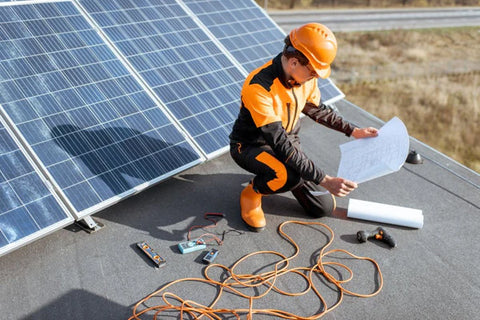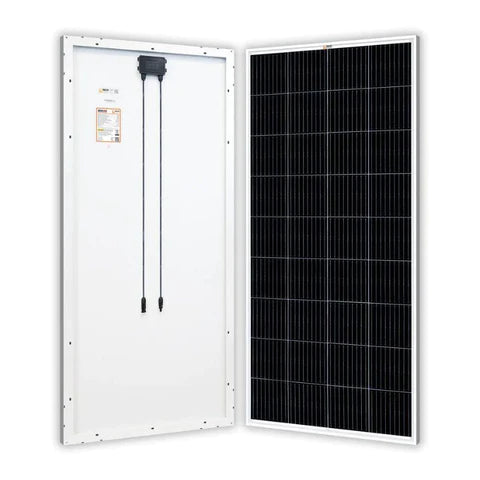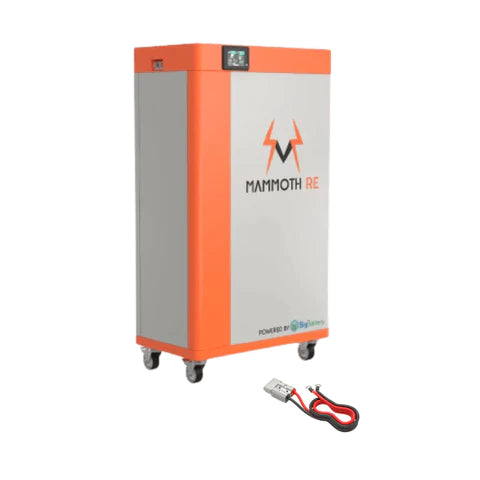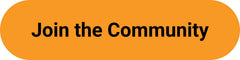Amp Wire Gauge (AWG) Calculator - ShopSolar.com

# Amp Wire Gauge Calculator

You may have heard of an amp wire gauge calculator without knowing what it entails. An amp wire gauge (AWG) calculator determines the wire size you need for an electrical installation. It is essential for solar panel users like yourself and can also be used to do other electrical jobs.

When you install solar panels in your home, basic electrical knowledge allows you to do the job yourself confidently. An amp wire gauge calculator is of great use to the avid DIYer. So keep reading to understand what it is and how to use it.

Input Values Result
Source Voltage:

120
240
480

Number of Phases: Single-Phase
3-Phase
*Amperes:
One Way Distance (feet):
Allowable Voltage Drop: 3% of source
5% of source
Volts
Wire Size

Copper

Aluminum

## What Is an Amp Wire Gauge Calculator?Every wire gauge (AWG) has a maximum current limit it can handle. And if you exceed the maximum capacity, you risk overheating, damage, and possibly even fire.

An amp wire gauge (AWG) calculator determines the wire size you need for connecting your solar panels.

### Why Do You Need an Amp Wire Gauge Calculator?

You must use the correct wire size when installing solar panels. But why?

Most residential use solar systems are compatible with wires between 8 and 14 gauges. However, this depends on the precise amps and watts of the solar system. So, different solar panel systems need different wiring sizes.

Using the wrong size wires (wires that are too small) will cause voltage drops that result in the loss of potential solar energy. But that’s not all. It can also be a fire hazard due to overheating. Nobody wants to waste power or take that fire risk, so selecting the correct wire size is crucial.#### Important Electrical Terms

Before we go any further, let’s address some basic electrical terms you’ll see when working with solar panels.

Amps: Amps are short for amperes and are units of electrical current.

Watts: Watts measures the rate of energy flow in an electrical system.

Volts: Voltage measures the electrical potential between two points in an electrical circuit.

You can convert amps to milliamps or, if necessary, convert amps to kva. Understanding these terms also allows you to calculate one when only the other metrics are available, for example, convert amps to volts.

## Calculate Amp Wire Gauge for a Solar Panel

Amp wire gauges refer to the size of the wires. The smaller the gauge, the bigger the wire, and the more amp capacity it has.

Solar wires come in standard sizes according to the American Wire Gauge. A 10-gauge solar wire can handle up to 30 amps, a 12-gauge can handle 20 amps, and a 14-gauge can handle 15 amps. When unsure, a 12 AWG cable is usually a safe bet.

### How to Use an Amp Wire Gauge Calculator

Most residential use solar PV panels are 12 volts. Let’s say you’ve just purchased a 200-watt 12v solar panel. And you want to know what size solar wire to connect it up. With our simple amp wire gauge calculator, you’ll maximize your solar panel energy and minimize the risk.The amperage decides the minimum AWG you have to get based on a 2 percent drop in volts. Just as you can convert amps to watts, you can also do the reverse if you know what the watts are.

Side note: Understanding amps is also useful when calculating your battery’s discharge time with an amp-hour calculator.You already know the watts, which enables you to calculate the amperage with a simple amp calculator.

• Divide your solar panel’s wattage by the voltage to determine the amps. Amps = Watts/volts = 200 watts/12 volts = 16.66 amps.

Next, you must apply the 80% National Electric Code (NEC) rule. According to this safety rule, the calculated amps represent 80% of the amp capacity of the wire you need to use. This safety measure means you’ll choose a slightly bigger wire, minimizing the fire risk we discussed earlier.

• To apply this rule, multiply the calculated amps ( in this case, 16.66) by 1.25 to get the minimum amp capacity.

Min. Am Capacity = Calculated Amps × 1.25

16.66 x 1.25 = 20,82.

This answer means that the wire gauge you use must be able to handle just over 20 amps. A 12 AWG solar cable has an amp capacity of 20 with a 2% voltage drop. Therefore, you can safely use a 12 gauge wire to connect your 200-watt 12-volt solar panel.

## Conclusion

An amp wire gauge calculator is an invaluable tool for solar panel enthusiasts. And just like all electrical calculations, it’s not that complicated once you understand how to do it and why it matters.

You can find all of the we have created in order to help you plan, size and install your own solar power system here.